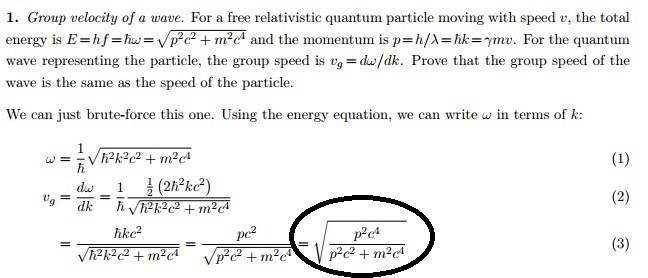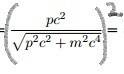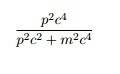# Can someone give me a hand with large root here

• lioric

#### liorici get the differentiation
the halves cancel the 2 the h bar cancels the h bar square
and to get rid of the root in the denominator the entire thing is squared
But I cannot understand where the huge square root came from at the end where I circled.
Can someone help me here

##a = \sqrt{a^2}## for positive ##a## and ##\frac{a}{\sqrt{b}} = \frac{\sqrt{a^2}}{\sqrt{b}} = \sqrt{\frac{a^2}{b}}##

##a = \sqrt{a^2}## for positive ##a## and ##\frac{a}{\sqrt{b}} = \frac{\sqrt{a^2}}{\sqrt{b}} = \sqrt{\frac{a^2}{b}}##

Ok i understand what you are saying.
But what if we square the entire thingAnd the root and the square gets canceled like thisOk i understand what you are saying.
But what if we square the entire thing
View attachment 96288
And the root and the square gets canceled like this
View attachment 96289
Exactly. If
$$\left( \frac{pc^2}{\sqrt{p^2c^2 + m^2 c^4}} \right)^2 = \frac{p^2c^4}{p^2c^2 + m^2 c^4}$$
then
$$\frac{pc^2}{\sqrt{p^2c^2 + m^2 c^4}} = \sqrt{\frac{p^2c^4}{p^2c^2 + m^2 c^4}}$$
which is what you have in original text.

You don't square the entire quotient. Set ##a=pc^2## and ##b = p^2c^2+p^2m^4##.

Exactly. If
$$\left( \frac{pc^2}{\sqrt{p^2c^2 + m^2 c^4}} \right)^2 = \frac{p^2c^4}{p^2c^2 + m^2 c^4}$$
then
$$\frac{pc^2}{\sqrt{p^2c^2 + m^2 c^4}} = \sqrt{\frac{p^2c^4}{p^2c^2 + m^2 c^4}}$$
which is what you have in original text.

Ok if one can get rid of the huge square root why keep it.
I mean as you said in the first part, why is the solution choosing to keep the huge root if we can choose to remove it

Ok if one can get rid of the huge square root why keep it.
I mean as you said in the first part, why is the solution choosing to keep the huge root if we can choose to remove it
It is a question of preference. Some things can be easier to see if all the terms are on the same footing (e.g., all under the square root), but where to stop simplifying can be a matter of taste.

It is a question of preference. Some things can be easier to see if all the terms are on the same footing (e.g., all under the square root), but where to stop simplifying can be a matter of taste.
Thank you very much
I'll try it both ways once I get a hang of things
Thank you very very much